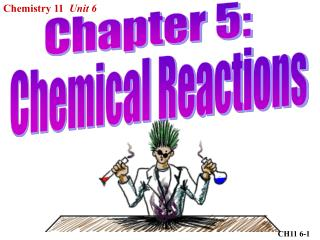DownloadDownload PresentationCH11 6-1

# CH11 6-1

Download Presentation## CH11 6-1

- - - - - - - - - - - - - - - - - - - - - - - - - - - E N D - - - - - - - - - - - - - - - - - - - - - - - - - - -
##### Presentation Transcript

1. Chemistry 11 Unit 6 Chapter 5: Chemical Reactions CH11 6-1

2. Chemical Equations • Chemical equations describe chemical reactions Ca(s) + 2H2O(l) H2(g) + Ca(OH)2(s) Ca(s) + 2H2O(l) H2(g) + Ca(OH)2(s) Ca(s) + 2H2O(l) H2(g) + Ca(OH)2(s) Ca(s) + 2H2O(l) H2(g) + Ca(OH)2(s) Ca(s) + 2H2O(l) H2(g) + Ca(OH)2(s) Ca(s) + 2H2O(l) H2(g) + Ca(OH)2(s) Reactants: What you start with in the reaction Products: What is produced by the reaction Formulas: represent chemicals Subscripts: represent phases: (s) solid, (l) liquid, (g) gas, (aq) aqueous – dissolved in water Coefficients: used to balance the equation • Chemical Equations: tell us amounts, state, and ratios of atoms in the reactants and products CH11 6-2

3. Balancing Equations • Remember the “Law of Conservation of Mass” • Write correct formulas for the reactants and products H2(g) + O2(g) H2O(l) • Check each type of atom to see if it is equal on both sides of the equation. Add coefficients to balance the atoms. H2(g) + O2(g) H2O(l) H2(g) + O2(g) 2H2O(l) 2H2(g) + O2(g) 2H2O(l) 2 H 2 O 2 H, 1 O 2 H 2 O 4 H, 2 O • Recheck each atom and add additional coefficients if necessary. (repeat this step until no more coefficients are required) 4 H 2 O 4 H, 2 O 2H2(g) + O2(g) 2H2O(l) CH11 6-3

4. Try this: Zn + HNO3 Zn(NO3) 2 + H2 Zn + 2HNO3 Zn(NO3) 2 + H2 Total atoms of each element: Zn H N O Zn H N O 1 1 1 3 1 2 2 6 Are they balanced?  × × ×  × × × Add a coefficient to HNO3 and check again: 1 2 2 6 1 2 2 6 Are they balanced?         Is that your final answer? Zn + 2HNO3 Zn(NO3) 2 + H2 CH11 6-4

5. Tips for Balancing Equations • Remember the following elements exist as diatomic molecules: H2 N2 O2 F2 Cl2 Br2 I2 At2 • Balance atoms which occur in only one molecule on each side of the equation first. • Balance groups of atoms when possible (SO42-, NO3-, NH4+,…) • Balance atoms in elemental form last (O2, Cl2, Fe,…) • Always try to be systematic PbO2 + HBr  H2O + PbBr 2 + Br2 e.g. • Pb already balanced • Balance O • Balance H • Balance Br Always check your answer!!! CH11 6-5

6. 2 2 4 Na2O2 + H2O  NaOH + O2 e.g. • Balance Na • Balance H • Balance O (use a fraction) • Remove fraction (multiply both sides of equation by 2) 3 2 3 Al2(SO4)3 + Ba(NO3)2 Al(NO3)3 + BaSO4 e.g. • Balance Al • Balance SO4 • Balance Ba • NO3 already balanced CHEM11 6-6

7. Using Algebra to Balance Equations Cu + HNO3  Cu(NO3)2 + NO + H2O A B C D E (Cu) A = C (H) B = 2E (N) B = 2C + D (O) 3B = 6C + D + E 3(N) - (O): 3B = 6C + 3D - (3B = 6C + D + E) 0 = 0 + 2D + E E = 2D Let E = 1, then B = 2, D = ½ CH11 6-7

8. From (N): B = 2C + D 2 = 2C + ½ 3/2 = 2C C = ¾ From (Cu): A = C = ¾ A B C D E ¾ 2 ¾ ½ 1 3 8 3 2 4 3 Cu + 8 HNO3  3 Cu(NO3)2 + 2 NO + 4 H2O CH11 6-8